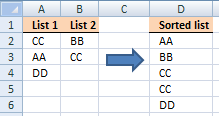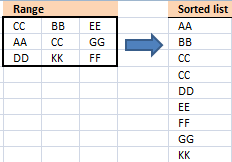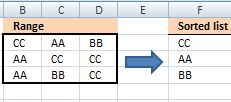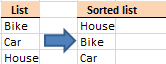### Create a new sorted list from a column using array formulaSort a column alphabetically

Table of Contents Sort a column using array formula Two columns sorting by the second column Sort alphanumeric values I […]

Create a unique distinct alphabetically sorted list

The array formula in cell D3 extracts unique distinct values sorted A to Z, from column B to column D. […]

### Create a new sorted list from two columns using array formulaSort text cells alphabetically from two columns

Table of Contents Sort text from two columns combined (array formula) Sort text from multiple cell ranges combined (user defined […]

### Create a new sorted list from a range using array formulaSort a range from A to Z [Array formula]

Question: How do I sort a range alphabetically using excel array formula? Answer: Cell range \$B\$2:\$E\$5 contains text values in random […]

Extract a unique distinct list sorted alphabetically and ignore blanks from a range

This is an answer to a question in this blog post: Extract a unique distinct list sorted from A-Z from […]

### MiscSort a range based on value frequency

The formula in cell B8 extracts a list sorted based on frequency. Array formula in B8: =TEXTJOIN("", TRUE, IF(MIN(IF((MAX(IF(COUNTIF(\$B\$7:B7, \$B\$2:\$E\$5)=0, […]Sort text values by length

Array formula in B2: =INDEX(\$B\$3:\$B\$20, MATCH(LARGE(LEN(\$B\$3:\$B\$20), ROWS(\$A\$1:A1)), LEN(\$B\$3:\$B\$20)*(COUNTIF(\$F\$2:F2, \$B\$3:\$B\$20)<COUNTIF(\$B\$3:\$B\$20, \$B\$3:\$B\$20)), 0)) copied down as far as needed. To enter an […]

Split values equally into groups

Question: How do I divide values equally into groups (3 lists or less)? This post shows you two different approaches, […]

### Dates

Sort dates within a date range

Array formula in D5: =SMALL(IF((\$B\$3:\$B\$12<=\$E\$3)*(\$B\$3:\$B\$12>=\$E\$2), \$B\$3:\$B\$12, "A"), ROWS(\$A\$1:A1)) How to create an array formula Copy array formula Select cell D5 […]